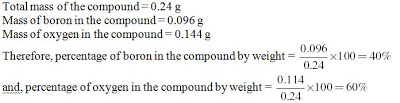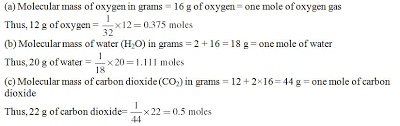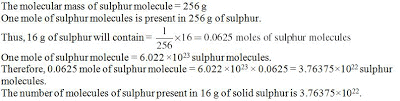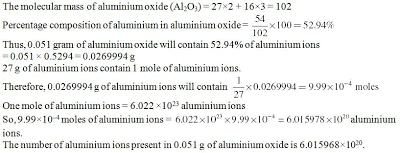# Atoms and Molecules - NCERT Solution, Class 9 Science Notes - Class 9

## Class 9: Atoms and Molecules - NCERT Solution, Class 9 Science Notes - Class 9

The document Atoms and Molecules - NCERT Solution, Class 9 Science Notes - Class 9 is a part of Class 9 category.
All you need of Class 9 at this link: Class 9
NCERT Exercises, Pages 43 - 44

1. A 0.24 g sample of compound of oxygen and boron was found by analysis to contain 0.096 g of boron and 0.144 g of oxygen. Calculate the percentage composition of the compound by weight.
Solution:(click solution for a larger image of the solution)

2. When 3.0 g of carbon is burnt in 8.00 g oxygen, 11.00 g of carbon dioxide is produced. What mass of carbon dioxide will be formed when 3.00 g of carbon is burnt in 50.00 g of oxygen?
Solution:
3.0 g of carbon combines with 8.0 g of oxygen to give 11.0 of carbon dioxide.
Thus, by the law of definite proportions, when 3.00 g of carbon is burnt in 50.00 g of oxygen, only 8.00 g of oxygen will be used to produce 11.00 gram of carbon dioxide. The remaining 42.00 g of oxygen will remain as is.

3. What are polyatomic ions? Give examples.
Solution: Polyatomic ions are those ions that contain more than one atom. These atoms can be of the same type of different type. Some examples of polyatomic ions are NO3-,NH4+,OH-, SO42-, and SO32-.

4. Write the chemical formulae of the following.
(a) Magnesium chloride
(b) Calcium oxide
(c) Copper nitrate
(d) Aluminium chloride
(e) Calcium carbonate.
Solution:
(a) MgCl2
(b) CaO
(c) Cu(NO3)2
(d) AlCl3
(e) CaCO3

5. Give the names of the elements present in the following compounds.
(a) Quick lime
(b) Hydrogen bromide
(c) Baking powder
(d) Potassium sulphate.
Solution:
(a) Calcium and oxygen
(b) Hydrogen and bromine
(c) Sodium, hydrogen, carbon, and oxygen
(d) Potassium, sulphur, and oxygen

6. Calculate the molar mass of the following substances.
(a) Ethyne, C2H2
(b) Sulphur molecule, S8
(c) Phosphorus molecule, P4 (Atomic mass of phosphorus = 31)
(d) Hydrochloric acid, HCl
(e) Nitric acid, HNO3
Solution:
(a) Molecular mass of ethyne, C2H2 = 2×12u + 2×u = 26u
(b) Molecular mass of sulphur molecule, S8 = 8×32u = 256u
(c) Molecular mass of phosphorus molecule, P4 = 4×31u = 124u
(d) Molecular mass of HCl = u + 35.5u = 36.5u
(e) Molecular mass of HNO3 = u + 14u + 3×16u = 63u

7. What is the mass of—
(a) 1 mole of nitrogen atoms?
(b) 4 moles of aluminium atoms (Atomic mass of aluminium = 27)?
(c) 10 moles of sodium sulphite (Na2SO3)?
Solution:
(a) Mass of one mole of nitrogen atoms = molecular mass of nitrogen atoms in grams = 14 g
(b) Mass of 4 moles of aluminium atoms = 4 × molecular mass of aluminum atoms in grams = 4 × 27 = 108
(c) Mass of 10 moles of sodium sulphite = 10 × molecular mass of sodium sulphite in grams
Molecular mass of sodium sulphite, Na2SO3 = 2×23 + 32 + 3×16 = 126 g
Thus, the mass of 10 moles of sodium sulphite = 10 × 126 = 1260 g

8. Convert into mole.
(a) 12 g of oxygen gas
(b) 20 g of water
(c) 22 g of carbon dioxide.
Solution:(click solution for a larger image of the solution)

9. What is the mass of:
(a) 0.2 mole of oxygen atoms?
(b) 0.5 mole of water molecules?
Solution:
(a) The mass of 1 mole of oxygen atoms = 16 g
Thus, the mass of 0.2 mole of oxygen atoms = 16 × 0.2 = 3.2 g
(b) The mass of 1 mole of water molecules = 18 g
Thus, the mass of 0.5 mole of water molecules = 18 × 0.5 = 9.0 g

10. Calculate the number of molecules of sulphur (S8) present in 16 g of solid sulphur.
Solution:(click solution for a larger image of the solution)

11. Calculate the number of aluminium ions present in 0.051 g of aluminium oxide.
(Hint: The mass of an ion is the same as that of an atom of the same element. Atomic mass of Al = 27 u)

Solution:The document Atoms and Molecules - NCERT Solution, Class 9 Science Notes - Class 9 is a part of Class 9 category.
All you need of Class 9 at this link: Class 9Use Code STAYHOME200 and get INR 200 additional OFF

Track your progress, build streaks, highlight & save important lessons and more!

,

,

,

,

,

,

,

,

,

,

,

,

,

,

,

,

,

,

,

,

,

,

,

,

;